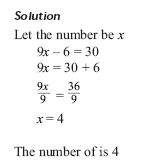Solving problems by forming equations

## Equation equality rule

The same quantity or number must be added to both sides of the equation without affecting the equality of the equation.

Solve 3x+2=7

Solution: subract 2 on both sides; 3x+2-2=7-2

Solve 3x-6=10

Solution: add 6 on both sides; 3x-6+6=10+6

Similarly the same quantity or number must be subtracted from both sides of the equation without affecting the equality of the equation.

Solve; 3x= 30

Solution: divide both sides with 3; 3x/3=30/3

Also divide or multiply both sides of the equation by the same quantity or number (except zero) without affecting the equality of the equation.

### Opposite operation rule

If an equation indicates an operation such as addition, subtraction, multiplication, or division, solve for the unknown by using the opposite operation.

For example, if the equation operation is addition, solve for the unknown by using subtraction.

Example

I think of a number, multiply it by 9 and then subtract 6 from the result. My answer is 30. What is the number?

Please study this solution and learn from it;